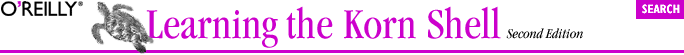home | O'Reilly's CD bookshelfs | FreeBSD | Linux | Cisco | Cisco Exam## 4.3. Compound Variables

```now="May 20 2001 19:44:57"        Assign current date to variable now
now.hour=19                       Set the hour
now.minute=44                     Set the minute
...
```

Note the use of the period in the variable's name. Here, now is called the parent variable, and it must exist (i.e., have a value) before you can assign a value to an individual component (such as hour or minute). To access a compound variable, you must enclose the variable's name in curly braces. If you don't, the period ends the shell's scan for the variable's name:

```\$ print \${now.hour}
19
\$ print \$now.hour
May 20 2001 19:44:57.hour
```

### 4.3.1. Compound Variable Assignment

Assigning to individual elements of a compound variable is tedious. In particular the requirement that the parent variable exist previously leads to an awkward programming style:

```person="John Q. Public"
person.firstname=John
person.initial=Q.
person.lastname=Public
```

Fortunately, you can use a compound assignment to do it all in one fell swoop:

```person=(firstname=John initial=Q. lastname=Public)
```

You can retrieve the value of either the entire variable, or a component, using print.

```\$ print \$person                                Simple print
( lastname=Public initial=Q. firstname=John )
\$ print -r "\$person"                           Print in full glory
(
lastname=Public
initial=Q.
firstname=John
)
\$ print \${person.initial}                      Print just the middle initial
Q.
```

NOTE: The order of the components is different from what was used in the initial assignment. This order depends upon how the Korn shell manages compound variables internally and cannot be controlled by the programmer.

A second assignment syntax exists, similar to the first:

```person=(typeset firstname=John initial=Q. lastname=Public ;
typeset -i age=42)
```

By using the typeset command, you can specify that a variable is a number instead of a string. Here, person.age is an integer variable. The rest remain strings. The typeset command and its options are presented in Chapter 6. (You can also use readonly to declare that a component variable cannot be changed.)

Just as you may use += to append to a regular variable, you can add components to a compound variable as well:

```person+= (typeset spouse=Jane)
```

A space is allowed after the = but not before. This is true for compound assignments with both = and +=.

The Korn shell has additional syntaxes for compound assignment that apply only to array variables; they are also discussed in Chapter 6.

Finally, we'll mention that the Korn shell has a special compound variable named .sh. The various components almost all relate to features we haven't covered yet, except \${.sh.version}, which tells you the version of the Korn shell that you have:

```\$ print \${.sh.version}
Version M 1993-12-28 m
```

We will see another component of .sh later in this chapter, and the other components are covered as we introduce the features they relate to.